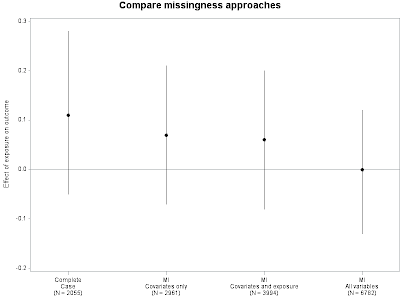## Wednesday, September 21, 2011

### Example 9.6: Model comparison plots (Completed)We often work in settings where the data set has a lot of missing data-- some missingness in the (many) covariates, some in the main exposure of interest, and still more in the outcome. (Nick describes this as "job security for statisticians").

Some analysts are leery of imputing anything at all, preferring to rely on the assumption that the data are missing completely at random. Others will use multiple imputation for covariates, but feel they should use "real" data for the exposure and outcome. Still others will impute the exposure but not the outcome. Theory and experiments suggest (Moons et al JCE 2006) that all missing data should be imputed. Depending on the imputation method, this may offer some protection against missing data that missing at random, more general than missing completely at random.

In one analysis, we decided to use each of these approaches and demonstrate the results that would be obtained. The data are shown below. The first column denotes the data used, the second has the effect on the mean and CI limits for the effect. How can we present these results clearly? We designed a graphic that requires some customization using either SAS or R but which makes the point elegantly.
`1 .11  1 -.051 .28 2 .07 2 .21 2 -.073 .06 3 -.083 .2 4 0 4 -.134 .12 `

SAS
The SAS version is shown below. (Click on it for a larger image.) To generate it, add a final column to the data, where the effect estimate is repeated but the other values are not. Then a basic plot can be created in proc gplot with the hiloc interpolation in the symbol statement and the overlay option in the plot statement. (See book section 5.3 and other blog entries for details.) Try the code without the axis statements to see what happens.
`data ke1;input datatype estimate meanval;cards;1 .11 .11 1 -.05 .1 .28 .2 .07 .072 .21 .2 -.07 .3 .06 .063 -.08 .3 .2 .4 0 04 -.13 .4 .12 .;;cards;run;symbol1 i=hiloc c=black v=none;symbol2 i=none v=dot h=1  c=black;axis1 minor=none order = (1 to 4 by 1)  value = (tick = 1 "Complete"              justify=c  "Case" justify = c "(N = 2055)"           tick=2 "MI" justify=c  "Covariates only"              justify=c "(N = 2961)"           tick=3 "MI" justify=c  "Covariates and exposure"              justify=c "(N = 3994)"           tick=4 "MI" justify=c  "All variables"              justify=c "(N = 6782)"           )  label = none  offset = (2 cm, 2 cm);axis2 minor=none order = (-.2 to .3 by .1)   label = (angle=90 "Effect of exposure on outcome");title "Compare missingness approaches";proc gplot data = ke1;plot (estimate meanval) * datatype /   overlay haxis=axis1 vref=0 vaxis=axis2;run;quit;`

The two axis statements make the plot work. The axis1 statement uses the value option to hand-write the labels describing the data sets. Note that the justify = c causes a new line to be started. The offset option adds a little space to the left and right of the data. The axis2 statement specifies the range and label for the vertical axis. The extra symbol statement and the overlay option just plot the dots that call attention to the effect estimates-- otherwise they would show just a small crossbar at the effect.

The plot suggests that as more observations are included and the multiple imputation gains accuracy the effect attenuates and the standard errors decrease.

R

In R we create the equivalent plot in multiple steps, first by creating an empty plot of the correct size then iterating through each of the lines. As with the SAS approach, a little manipulation of the raw data is required.
`n = c(2055, 2961, 3994, 6782)labels = c("Complete Case", "MI\ncovariates only",           "MI\ncovariates and exposure",           "MI\nall variables")est =    c(0.11,  0.07,  0.06,  0)lower = c(-0.05, -0.07, -0.08, -0.13)upper =  c(0.28,  0.21,  0.20,  0.12)plot(c(0.5, 4.5), c(min(lower)-.10, max(upper)), type="n", xlab="",   xaxt="n", ylab="Effect of exposure on outcome")title("Compare missingness approaches")for (i in 1:length(n)) {  points(i, est[i])  lines(c(i,i), c(lower[i], upper[i]))  stringval = paste(labels[i],"\n(N=",n[i],")")  text(i, min(lower) - .05, stringval, cex=.6)}abline(h=0, lty=2)`

The resulting plot is shown at the top. As opposed to the SAS approach, more of the figure can be defined using the data. For example, the y-axis values are determined from the minimum and maximum values to plot.Note: a draft of this entry was published accidentally. Many apologies. --Ken